# High School Math : Understanding Normal Distributions

## Example Questions

### Example Question #11 : Normal Distribution

Which of the following populations has a precisely normal distribution?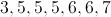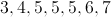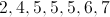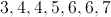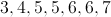Explanation:

A normal distribution is one in which the values are evenly distributed both above and below the mean.  A population has a precisely normal distribution if the mean, mode, and median are all equal.  For the population of 3,4,5,5,5,6,7, the mean, mode, and median are all 5.

### Example Question #12 : Normal Distribution

If a population has a normal distribution, the number of values within one positive standard deviation of the mean will be . . .

equal to the number of values within two negative standard deviations of the mean

fewer than 20% of all values in the population

at least 40% of all values in the population

fewer than the number of values within one negative standard deviation of the mean

equal to the number of values within one negative standard deviation of the mean